## Introduction:

Esterline Research and Design (ERD) is a frequency control company that designs and manufactures oscillators and test and measurement gear for the oscillator industry. As a consequence, we also manufacture high-high end counter products to qualify and test those time bases.

Instead of using reciprocal RF counters at the output frequency of the DUT (device under test), we use the heterodyne method to increase resolution and increase channel to channel isolation by mixing down to audio frequencies instead of counting RF.

The following will focus on how the heterodyne method dramatically increases counter resolution.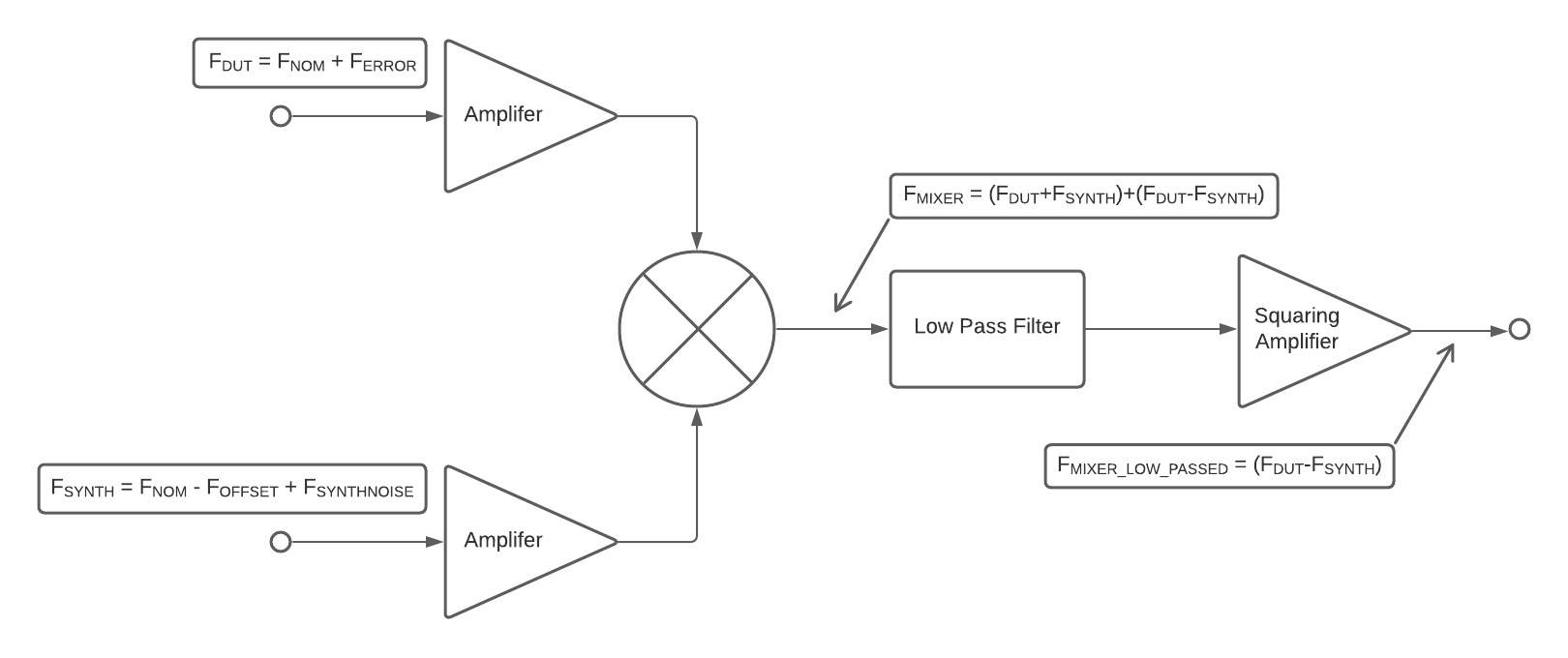Figure 1: Down converter block diagram

Frequency mixing and down conversion (heterodyne techniques) are a common method used by frequency control engineers to increase the resolution of their measurements. A common objection from those not familiar with this technique is that "dividing down" the frequency also squashes down the error, which is tantamount to a wash. This would be true if we were talking about dividing down the frequency, but mixing down and dividing down are different processes which achieve different results. Let's take a closer look at the math involved.

## Native Counter Resolution:

Our ERD frequency counters have a native resolution of 12.5 ppb/s (parts per billion per second) due to the counter's microprocessor running at 80MHz.

Say your desired measurement resolution of a 10 MHz signal is 1 ppb. To find that resolution in terms of Hz, we multiply by (1/1E9).So we need a counter with a native resolution of at least 0.01 Hz in one second. Calculating the counter's native resolution in Hz reveals the problem with this:This is worse than our desired resolution by greater than an order of magnitude, making it unsuitable for our purpose. But what happens if we mix down?

## Down Conversion:

If we mix the signal down to 1 KHz, there are two important elements we must consider.

1.) What is our counter resolution at 1 KHz?

2.) What is the content of the 1 KHz Intermediate Frequency (IF)?

First, let's look at the resolution.This is obviously a far greater resolution than can be counted on a 10 MHz signal with the counter's native resolution. And this is where the aforementioned objection most often comes into play: "But you need to multiply that back up in order to get your measurement."  This isn't true for a mixed-down signal because we still capture the raw Δf of the DUT.

Let's take a deeper dive into why. As can be seen in Figure 1, we mix the DUT against a low-noise synthesizer. The synthesizer selected should have an Allen Deviation at least an order of magnitude better than that of the DUT. Front-end amplifiers are implemented on both the DUT and synthesizer inputs to provide the correct power level to the chosen mixer.

## DUT Frequency:

The equation for the frequency of the DUT can be expressed in the following manner: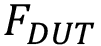is the frequency of the signal source under test.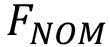is the nominal frequency of the signal source.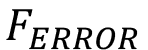is the signal source's error from the nominal frequency for all sources of error (temperature, aging, noise, etc).

(Note thatcan be signed positive or negative).

Further note thatis the deviation from the nominal frequency we are trying to measure. The synthesizer is locked to our system's standard, so its error from nominal is assumed to be zero. There is some amount of noise on the synth, but it should be at least an order of magnitude better than the DUT, so we assume it to be negligible for our purposes.

## Synth Frequency:

The equation for the frequency of a synthesizer can be expressed in the following manner: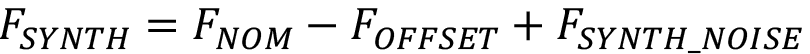is the frequency of the low-noise synthesizer we are mixing against.is the nominal frequency of the DUT.is the selected IF for the measurement.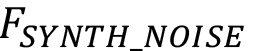is the noise of the synthesizer, which is negligible compared to the DUT noise.

Note that 1 KHz is the value we have chosen foras the IF for this example, but it is not the only frequency that can be chosen. It is necessary to chose an IF large enough such that the DUT will not move an appreciable percentage of the IF due to any source of frequency change. We have chosen 1/10 of the IF as our limit due to empirical observations and aberrant readings as you approach 50% of the IF.

## Mixing Calculations:

When we mix the Synth with the DUT, we obtain the sum and difference of the two signals: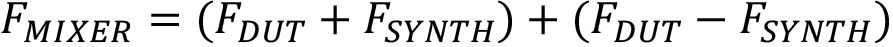As can be seen in Figure 1 the output is passed through a low pass filter with a cutoff frequency equal to the IF. The low pass filter strips off the sum portion of the solution, leaving the following:The low-passed signal is fed into a squaring amplifier to condition the signal for ease of counting in the microprocessor.

In order to better understand why the error of the DUT is preserved, let's make some substitutions from our earlier mathematical definitions ofand: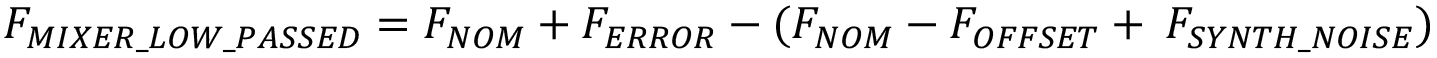We then distribute the negative sign:Collecting terms causes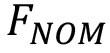to drop out: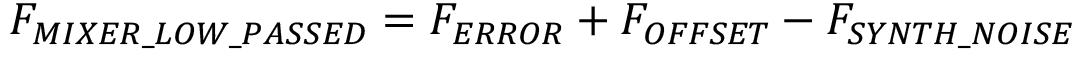Note thatis fixed. In this example, it is 1 KHz.Remember, we are assumingis negligible, leaving us with: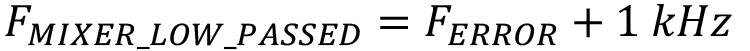Also note from before that when we measure the IF at this frequency with a 12.5 ppb/s counter, we have a resolution of 0.0000125 Hz.  In fact, when we use this method, your increase in resolution is equal to the mix-down factor. Selecting an IF of 1KHz with a 10MHz input is a mix-down factor of 10,000.Since we are measuring the DUT's deviation from nominal frequency, we can say: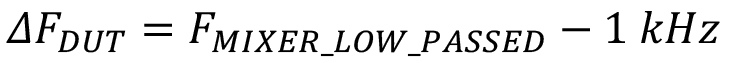## Conclusion:

This means that when we mix down to an IF of 1 KHz, we preserve the error magnitude from the original signal source. Therefore, the misconception about needing to multiply up is proven false, and the down conversion preserves the raw error present on the DUT.

Our down converter math returns, so to get the actual frequency measurement,must be added to. Sinceis signed, it can be either plus or minus, resulting in a measurement above or below.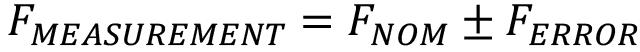ERD has optimized this concept to allow for the manufacture of test systems with thousands of channels and extremely low noise floors. We have incorporated this method into test systems which evaluate parameters such as frequency vs. temperature, aging, ADEV, and microjumps, etc. We supply these systems to the oscillator manufacturing industry with a customer base across the globe.